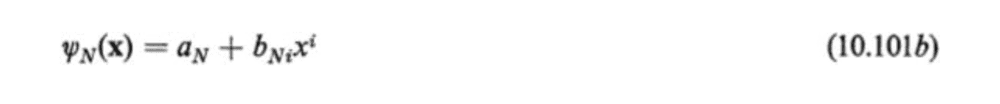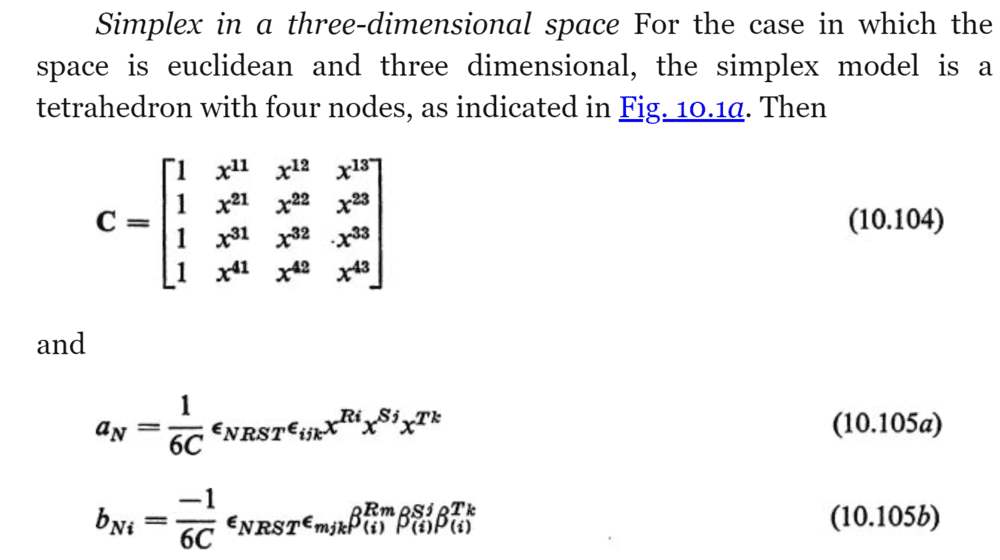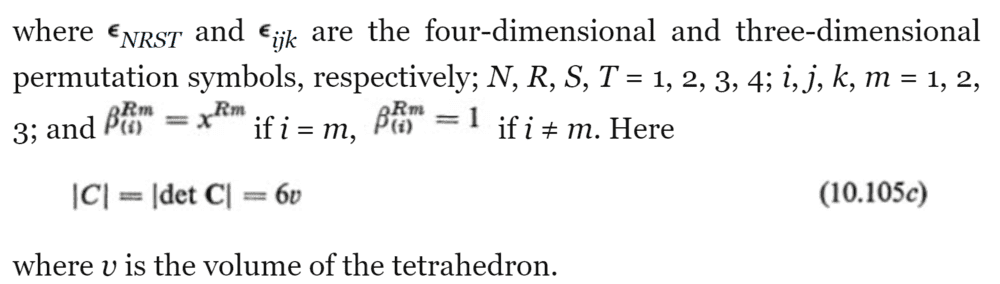# Tetrahedron Simplex Shape Functions in FEA

• doenn1616
In summary, the tetrahedron finite element method seems to produce incorrect results for points outside of the simplex.In summary, the tetrahedron finite element method seems to produce incorrect results for points outside of the simplex.f

#### doenn1616

TL;DR Summary
Have tested 2D triangular shape functions successfully but having trouble with 3D.
Hi, 2 part question trying to get tetrahedron Finite Element shape functions working: 1) How do I properly setup the shape coefficient matrix and 2) How do I build the coefficient quantities in the shape functions properly? ANY tips or corrections may unblock me and would be of much value!

Following J.T. Oden's "Finite Elements of Nonlinear Continua" (McGraw Hill), general simplex:Tetrahedron Simplex:Permutation symbol steps and shape coefficient matrix (C) description:I attached the full Matlab test. I don't mind if this works in Matlab or anything else, as long as I can understand. Flow of my Matlab:

Tetrahedron points. I read the ordering of these is critical so that the coefficient matrix is positive (not sure if this is true?):
Code:
p1 = [2,0,8]
p2 = [2,0,0]
p3 = [12,0,0]
p4 = [2,8,0]

Code:
points = buildPoints(p1,p2,p3,p4)      % Just organization of points
shapeCoeff = buildShapeCoeff(p1,p2,p3,p4)

First struggle:
Within buildShapeCoeff() I did this (Line 100) in order to get a positive determinant... Is this needed? I tried without and the bNi coefficient in 10.105b kept coming up zero?

shapeCoeffRes = shapeCoeffRes' % Transpose rowmajor versus colmajor

Lines 45 and 46 yield 1/6C or -1/6C as required:
Code:
aCoeffPrefix = buildACoeffPrefix(shapeCoeff)  %Line 45
bCoeffPrefix = buildBCoeffPrefix(shapeCoeff)   %Line 46

Then I try calculating aN and bNi (Figures 10.105a and 10.105b) with the following:

Code:
aVals = calcAVals(aCoeffPrefix, points) % Line 48

bVals = calcBVals(bCoeffPrefix, points) % Line 50

Finally at line 62 I try calculating Figure 10.101b. The result is not 0 but it is out of range of expectations. If ANY more detail is required, I can provide quickly.

#### Attachments

• FiniteElement_TXT_Extension.txt
7.8 KB · Views: 93
Last edited by a moderator:
There are some good books about the implementation of FEM in Matlab that can be helpful for you. Personally I recommend "The Finite Element Method Using Matlab" Y.W. Kwon. It features a Matlab code for 3D elements, among others.

Hi, I've bought several books on this and related topics including "Peter I. Kattan MATLAB Guide to Finite Elements An Interactive Approach" which I just found out has a download link here
https://extras.springer.com/2008/978-3-540-70697-7.zip

Does the book you mention cover details on 3D shape functions (of any order)? I looked in the sample PDFs and it includes ~3 pages on "Three Dimensional Frame Element"s on pages 265-268.

The Matlab book I have has functions on calculating the volume term (attached) and then goes straight into stiffness matrices. Looking to specifically get the interpolant step down.

(Oden's book's are nice since they show general formulas to generalize the shape functions to different simplex types and higher orders. If this thread leads to a dead-end, I'll just keep trying to experiment, ask smaller questions, or ask about a step I may be missing in this generalization instead.)

Other attachments:
My latest attempt is attached as well: FiniteElement_2021-04-07 Result val shows 23.6 regardless of test point, which I think is wrong. My FiniteElement_2D uses Oden's method and yields a final val of 35.285714285714285 which I think is correct. Other test points interpolate correctly as well.

#### Attachments

• TetrahedronElementVolume.txt
631 bytes · Views: 79
• FiniteElement_2021-04-07.txt
7.9 KB · Views: 90
• FiniteElement_2D.txt
1.9 KB · Views: 76